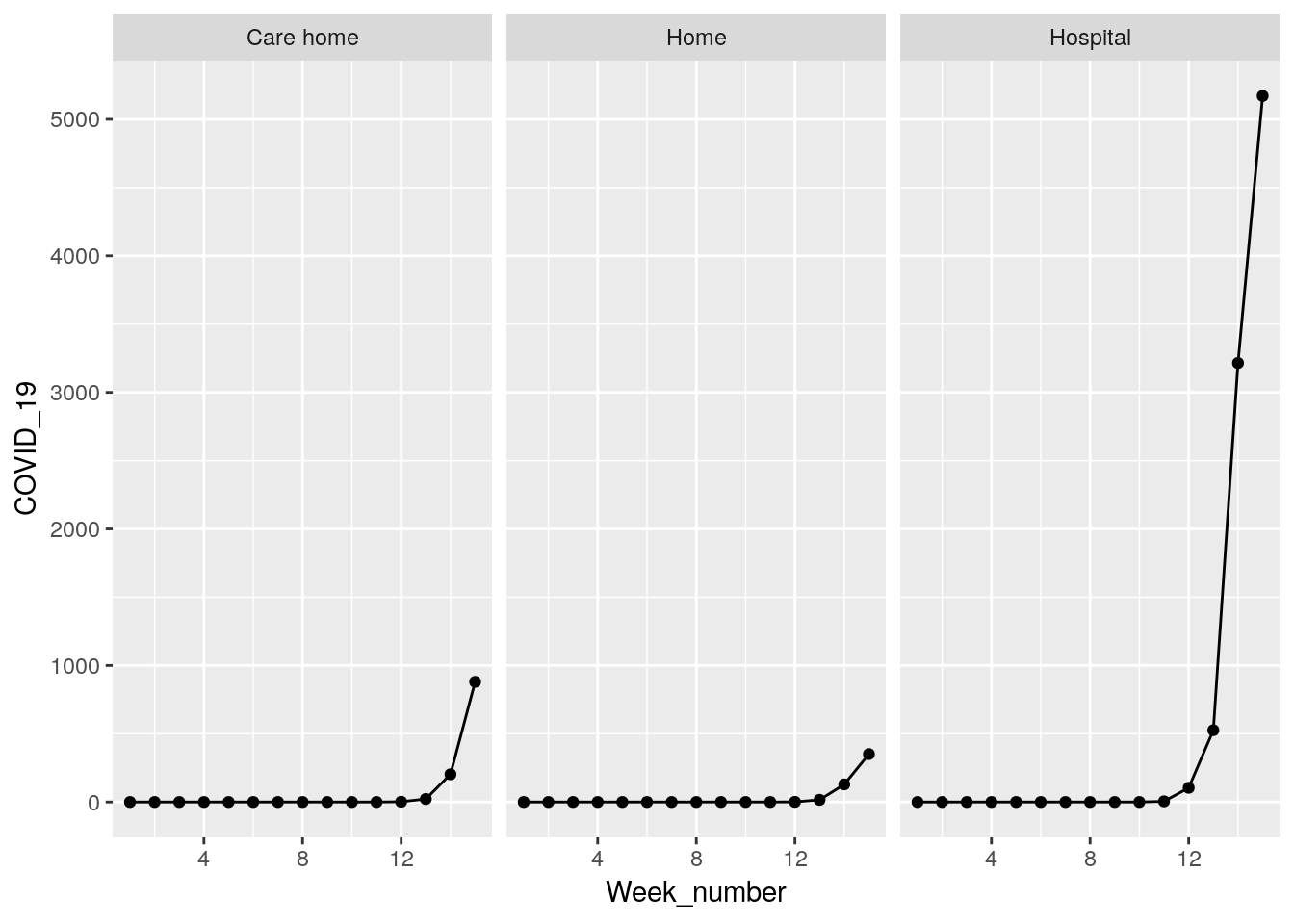## Load data from Office for national statistics: Occurrences

library(readxl)
sheet = "Occurrences - All data", skip = 2)

names(d) <- gsub(" ", "_", names(d))
d$Cause_of_death <-gsub(" ","_",d$Cause_of_death)
d  %>% group_by(Week_number, Cause_of_death, Place_of_death) %>% summarise(sum=sum(Number_of_deaths)) %>% pivot_wider(names_from ="Cause_of_death",values_from = "sum") %>% mutate(Other_causes=All_causes-COVID_19) -> deaths

## Deaths from all causes

deaths %>% filter(Place_of_death %in% c("Care home","Home","Hospital")) %>%
ggplot(aes(x=Week_number,y=All_causes)) + geom_point() + geom_line() + facet_wrap(~Place_of_death )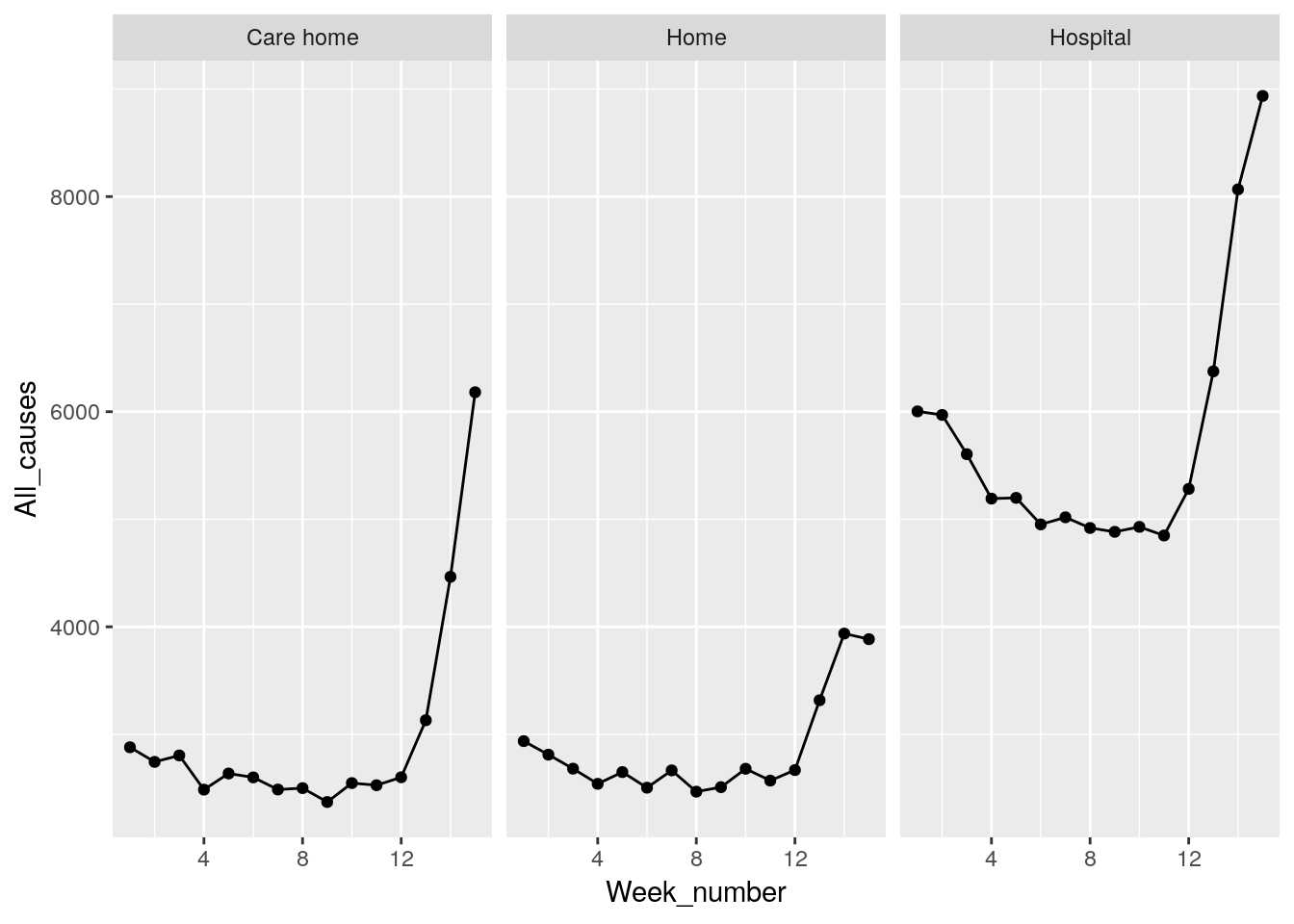## Deaths excluding Covid

deaths %>% filter(Place_of_death %in% c("Care home","Home","Hospital")) %>%
ggplot(aes(x=Week_number,y=Other_causes)) + geom_point() + geom_line() + facet_wrap(~Place_of_death )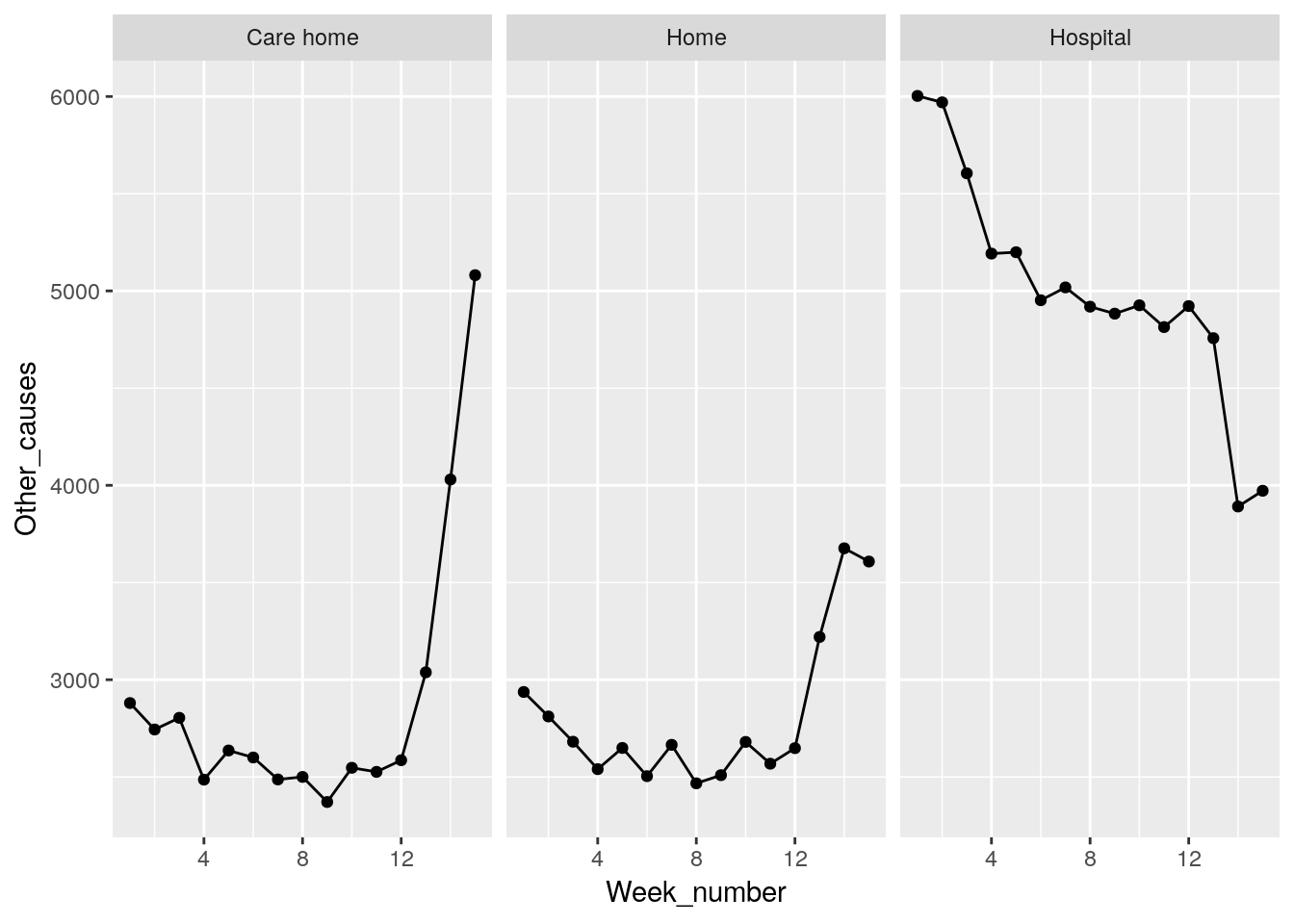## Deaths With Covid mentioned

deaths %>% filter(Place_of_death %in% c("Care home","Home","Hospital")) %>%
ggplot(aes(x=Week_number,y=COVID_19)) + geom_point() + geom_line() + facet_wrap(~Place_of_death )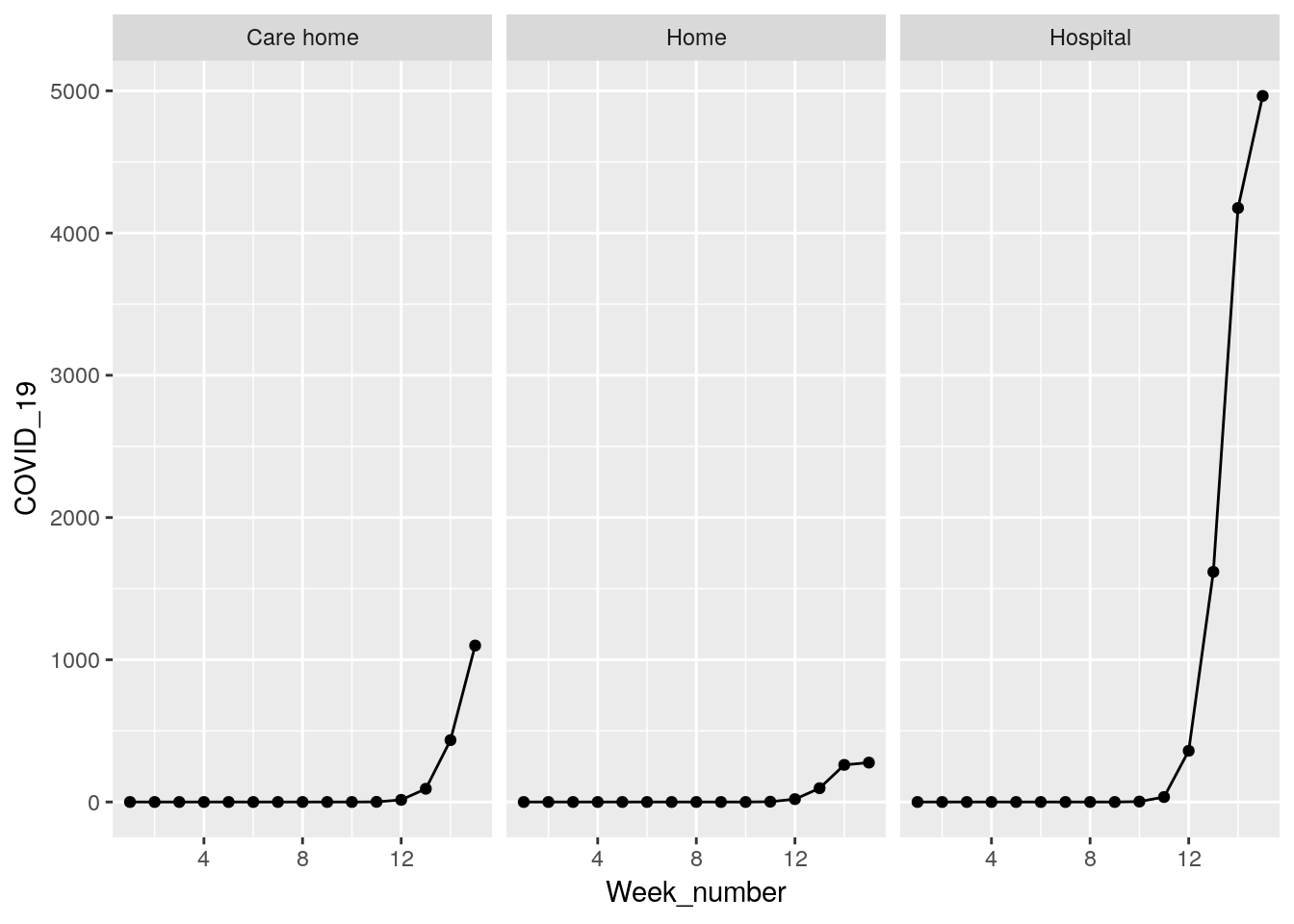## Using registrations data

library(readxl)
sheet = "Registrations - All data", skip = 2)

names(d) <- gsub(" ", "_", names(d))
d$Cause_of_death <-gsub(" ","_",d$Cause_of_death)
d  %>% group_by(Week_number, Cause_of_death, Place_of_death) %>% summarise(sum=sum(Number_of_deaths)) %>% pivot_wider(names_from ="Cause_of_death",values_from = "sum") %>% mutate(Other_causes=All_causes-COVID_19) -> deaths

## Deaths from all causes

deaths %>% filter(Place_of_death %in% c("Care home","Home","Hospital")) %>%
ggplot(aes(x=Week_number,y=All_causes)) + geom_point() + geom_line() + facet_wrap(~Place_of_death )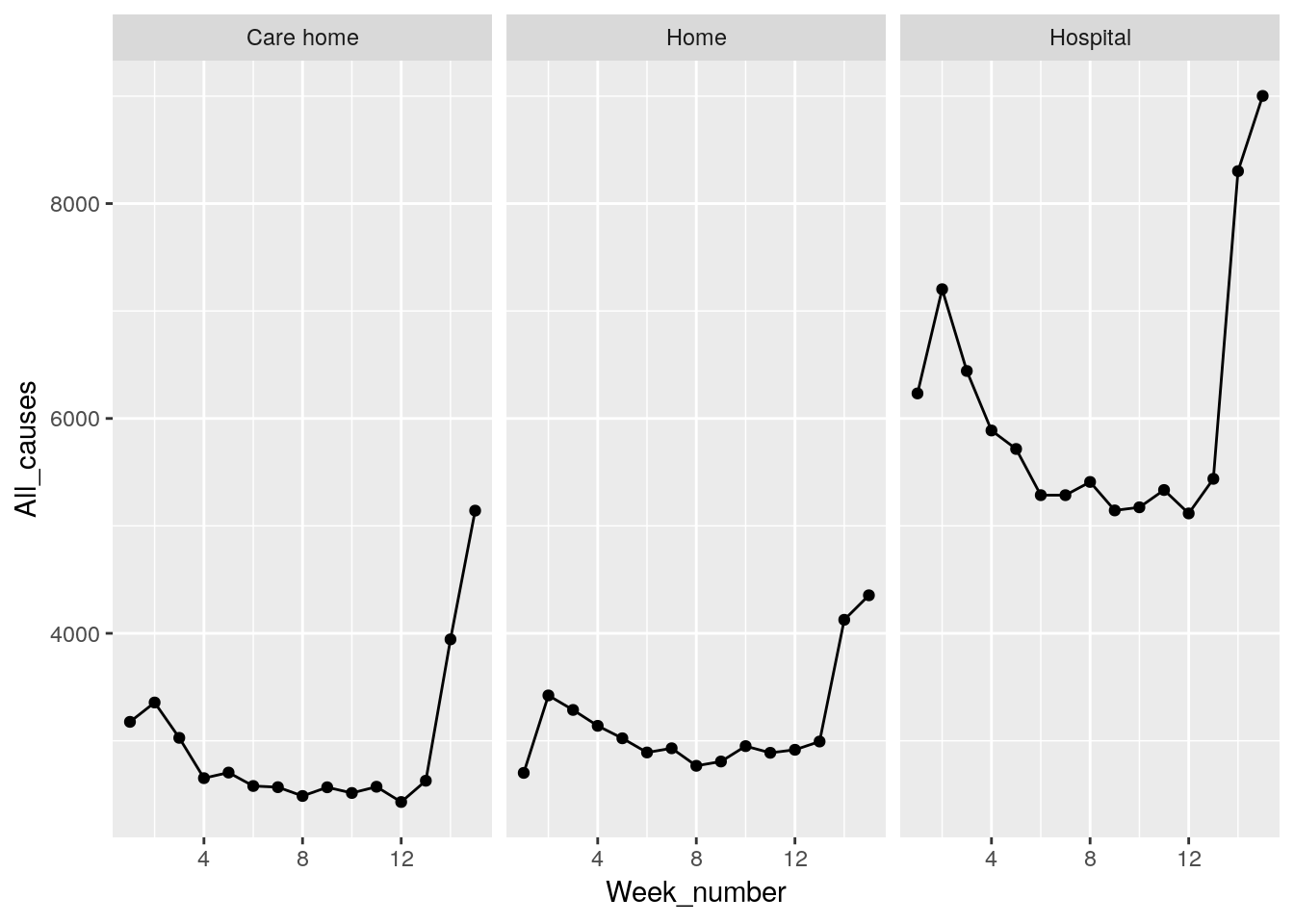## Deaths excluding Covid

deaths %>% filter(Place_of_death %in% c("Care home","Home","Hospital")) %>%
ggplot(aes(x=Week_number,y=Other_causes)) + geom_point() + geom_line() + facet_wrap(~Place_of_death )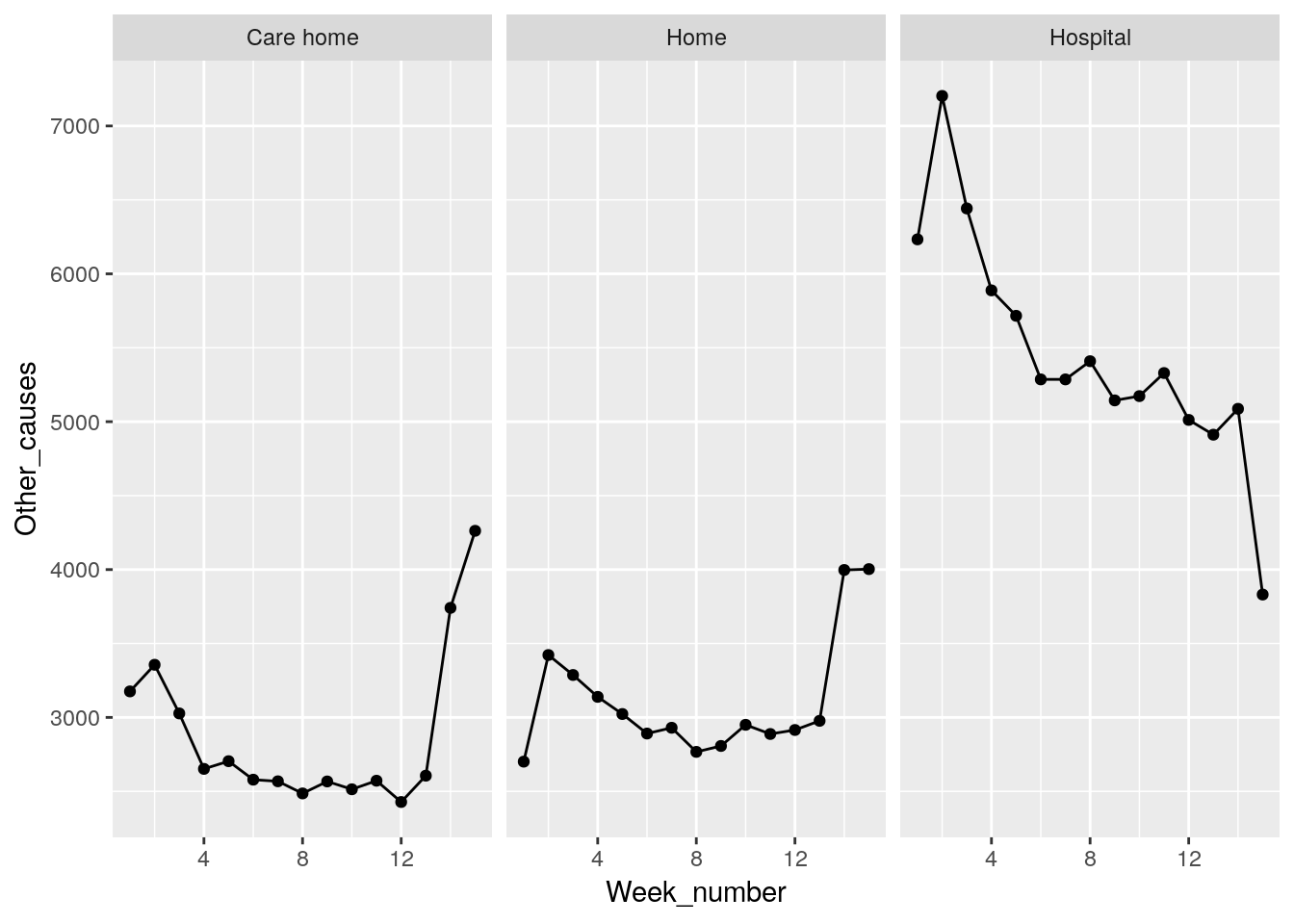## Deaths With Covid mentioned

deaths %>% filter(Place_of_death %in% c("Care home","Home","Hospital")) %>%
ggplot(aes(x=Week_number,y=COVID_19)) + geom_point() + geom_line() + facet_wrap(~Place_of_death )